# ISEE Upper Level Math : How to find an angle in a hexagon

## Example Questions

### Example Question #5 : Hexagons

If the sum ofangles in a hexagon is equal to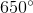, what is the value of the remaining sixth angle?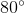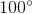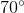Explanation:

The sum of the angles in a polygon can be found using the equation below, in which t is equal to the total sum of the angles, and n is equal to the number of sides.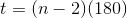Given that a hexagon has 6 angles, the total number of angles will be: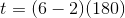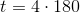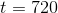Given that 5 of the angles have a sum of 650 degrees, we would subtract 650 from 720, resulting in 70 degrees.